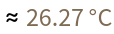#Function Repository Resource:

# WindChill

Calculate the effect of the wind on the temperature that humans perceive

Contributed by: Claudio Lobo Chaib Filho
 ResourceFunction["WindChill"][] gives the wind chill for the user's location. ResourceFunction["WindChill"][loc] gives the wind chill for a specific location loc. ResourceFunction["WindChill"][{t,ws}] gives the wind chill for a given temperature t and wind speed ws. ResourceFunction["WindChill"][…,form] gives the result in the desired output form form.

## Details and Options

Wind chill is the temperature perceived by humans, taking into account air temperature and wind speed. The function works with temperature values between -70 °C and 10 °C and values between 4.282 km/h and 400 km/h for wind speed.
The loc argument is a location in the form of an Entity, a GeoPosition or Here. This information comes from the nearest weather station to that location and the value may vary over time. A list of air temperature and wind speed information can be used in place of a location.
The form argument can be a temperature, a plot or an association giving both temperature and plot:
 "Temperature" the perceived temperature due to wind chill "Plot" a plot of the perceived temperature versus wind speed at the given air temperature "PropertyAssociation" all results as an Association
The default value of the form argument when not given is "Temperature".
If the temperature unit is not specified for the t argument, the input is considered degrees Celsius. Examples of degree units for temperature accepted by the function are:
 Quantity[-20,"DegreesCelsius"] degrees Celsius Quantity[-90,"DegreesFahrenheit"] degrees Fahrenheit Quantity[420,"DegreesRankine"] degrees Rankine Quantity[215,"Kelvins"] Kelvins -20 degrees Celsius
If the wind speed unit is not specified for the ws argument, it is considered kilometers per hour. Examples of input allowed for wind speed and how it is interpreted are:
 Quantity[30, "Kilometers"/"Hours"] kilometers per hour Quantity[20, “Miles"/"Hours”] miles per hour 15 kilometers per hour
ResourceFunction["WindChill"] accepts the option "ResultUnit" to specify the unit of the result. The accepted values are "DegreesFahrenheit", "DegreesCelsius", "DegreesRankine" and "Kelvins". By default, the unit is inherited from the inputs. If no unit is provided, "DegreesCelsius" is used.

## Examples

### Basic Examples

Using WindChill without arguments uses Here as the location:

 In:=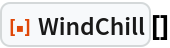Out=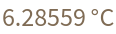Find the wind chill at a given location:

 In:=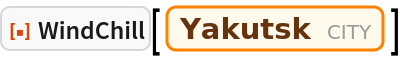Out=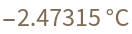This is equivalent to using "Temperature" as a second argument:

 In:=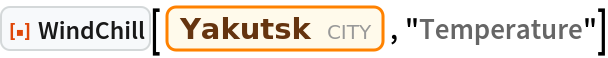Out=Use WindChill with a temperature and wind speed:

 In:=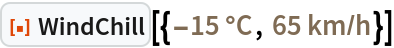Out=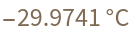### Scope

The air temperature can be given in degrees Fahrenheit. Note that the result has the same temperature units:

 In:=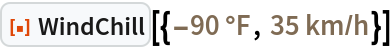Out=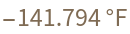If the temperature is not explicitly given a unit, it is interpreted as degrees Celsius:

 In:=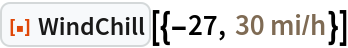Out=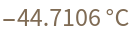If the wind speed is not explicitly given a unit, it is interpreted as kilometers per hour:

 In:=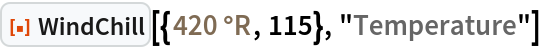Out=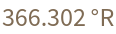Return a plot:

 In:=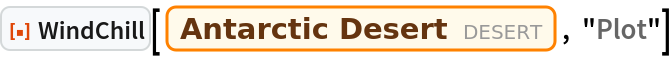Out=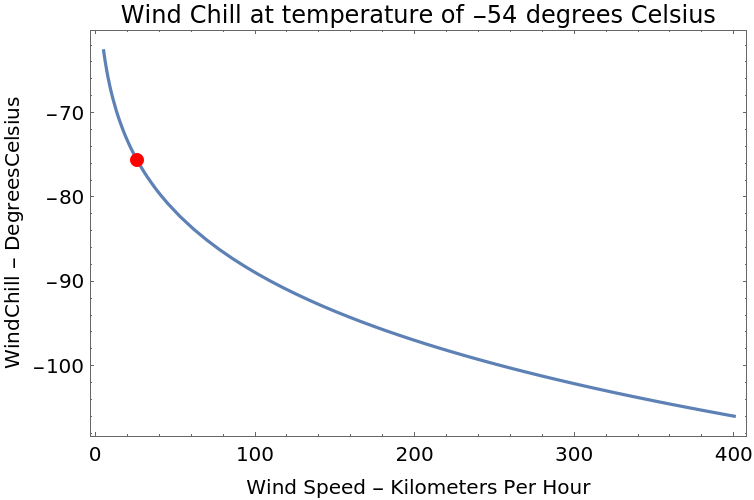Get an Association of results:

 In:=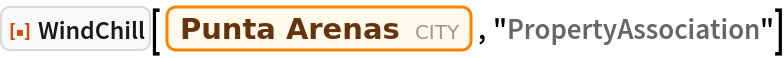Out=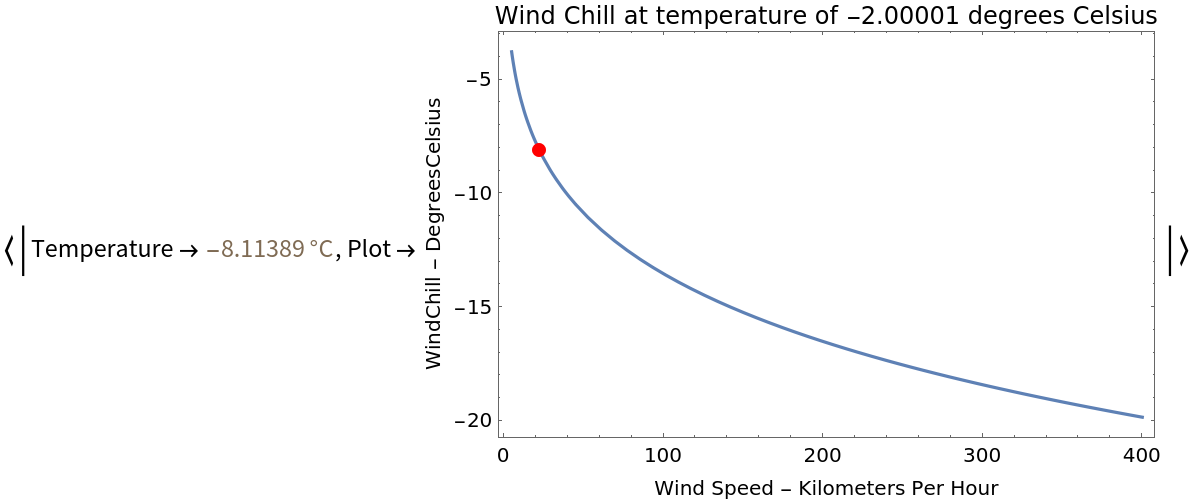The function can accept a GeoPosition instead of an Entity:

 In:=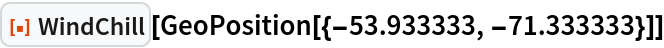Out=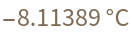### Options

The "ResultUnit" option specifies the temperature unit for the result:

 In:=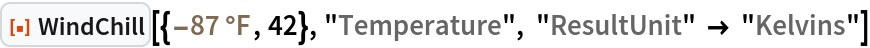Out=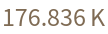By default, WindChill uses either the input units or degrees Celsius if the input is a location:

 In:=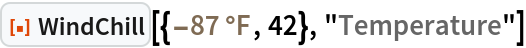Out=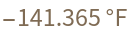In:=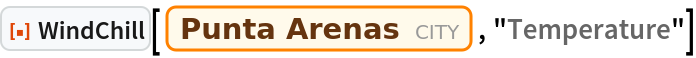Out=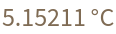Note that the "Plot" output is also changed in the plot when the option "ResultUnit" option is given:

 In:=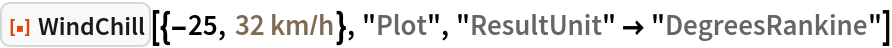Out=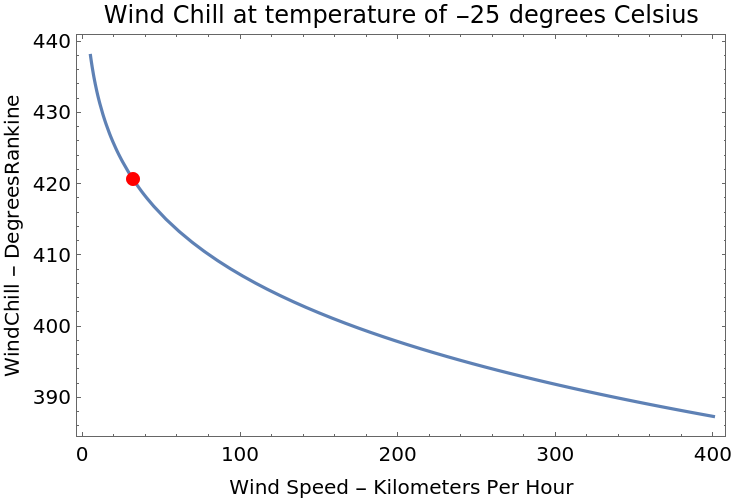### Possible Issues

Many times, the temperature of a specific location is outside the possible range for wind chill calculation. In these cases, the temperature of the result is provided directly by the weather station as wind chill; TildeTilde appears in the answer and a message is issued saying that the value is not accurate:

 In:=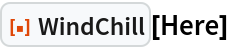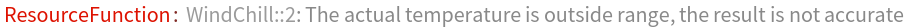Out=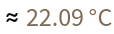Sometimes the nearest weather station to a specific location does not have air temperature, wind speed or wind chill data:

 In:=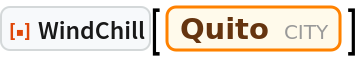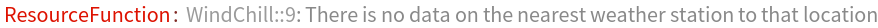Even if the unit of the input wind speed is different from kilometers per hour, the x axis in the plot remains in kilometers per hour:

 In:=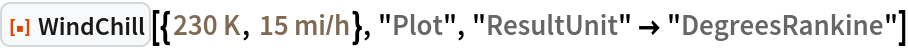Out=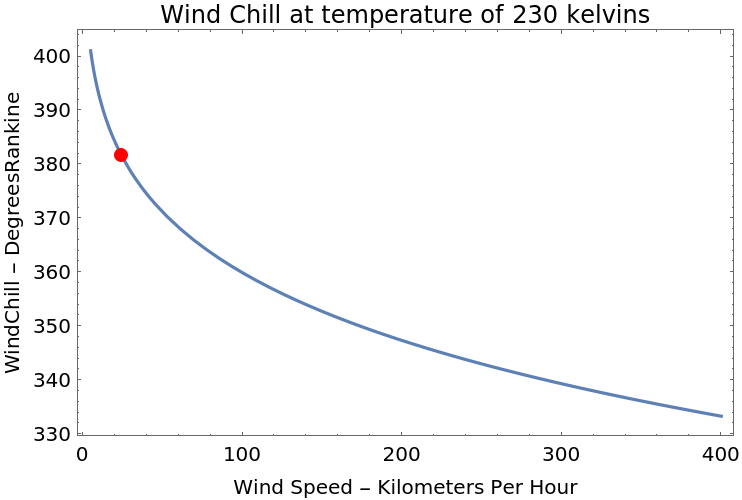The function only accepts temperature values between -70 °C and 10 °C and wind speed between 4.828 km/h and 400 km/h, so when a specific weather station provides temperature and wind speed values outside these limits, the function automatically acquires the value of the wind chill directly from the weather station itself:

 In:=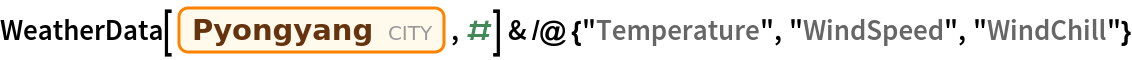Out=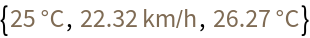In:=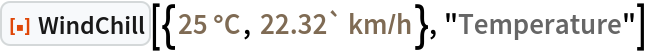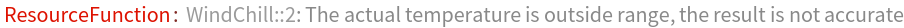Out=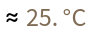In:=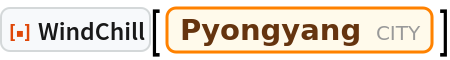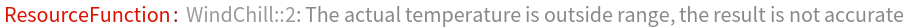Out=